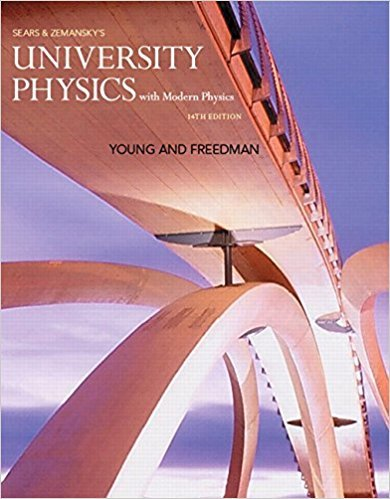×
×

# Solution: An astronaut has left the International SpaceISBN: 9780321973610 228

## Solution for problem 2.16 Chapter 2

University Physics with Modern Physics (1) | 14th Edition

• Textbook Solutions
• 2901 Step-by-step solutions solved by professors and subject experts
• Get 24/7 help from StudySoup virtual teaching assistantsUniversity Physics with Modern Physics (1) | 14th Edition

4 5 1 290 Reviews
23
2
Problem 2.16

An astronaut has left the International Space Station to test a new space scooter. Her partner measures the following velocity changes, each taking place in a 10-s interval. What are the magnitude, the algebraic sign, and the direction of the average acceleration in each interval? Assume that the positive direction is to the right. (a) At the beginning of the interval, the astronaut is moving toward the right along the x-axis at 15.0 m>s, and at the end of the interval she is moving toward the right at 5.0 m>s. (b) At the beginning she is moving toward the left at 5.0 m>s, and at the end she is moving toward the left at 15.0 m>s. (c) At the beginning she is moving toward the right at 15.0 m>s, and at the end she is moving toward the left at 15.0 m>s

Step-by-Step Solution:
Step 1 of 3

Physics 2080 Chapter 18: Laws of Thermodynamics January 19, 2016 Amanda Biddlecome 1) Zeroth Law of Thermodynamics -­‐Object...

Step 2 of 3

Step 3 of 3

##### ISBN: 9780321973610

This full solution covers the following key subjects: . This expansive textbook survival guide covers 44 chapters, and 4574 solutions. Since the solution to 2.16 from 2 chapter was answered, more than 232 students have viewed the full step-by-step answer. University Physics with Modern Physics (1) was written by and is associated to the ISBN: 9780321973610. This textbook survival guide was created for the textbook: University Physics with Modern Physics (1), edition: 14. The answer to “An astronaut has left the International Space Station to test a new space scooter. Her partner measures the following velocity changes, each taking place in a 10-s interval. What are the magnitude, the algebraic sign, and the direction of the average acceleration in each interval? Assume that the positive direction is to the right. (a) At the beginning of the interval, the astronaut is moving toward the right along the x-axis at 15.0 m>s, and at the end of the interval she is moving toward the right at 5.0 m>s. (b) At the beginning she is moving toward the left at 5.0 m>s, and at the end she is moving toward the left at 15.0 m>s. (c) At the beginning she is moving toward the right at 15.0 m>s, and at the end she is moving toward the left at 15.0 m>s” is broken down into a number of easy to follow steps, and 142 words. The full step-by-step solution to problem: 2.16 from chapter: 2 was answered by , our top Physics solution expert on 01/09/18, 07:46PM.

Unlock Textbook Solution